## Reactive Power and The Power Triangle:

We know that the average power dissipated is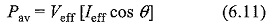From the impedance triangle shown in Fig. 6.4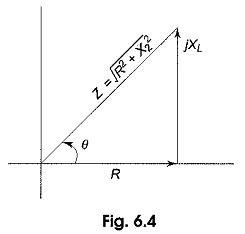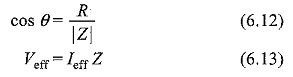If we substitute Eqs (6.12) and (6.13) in Eq. (6.11), we get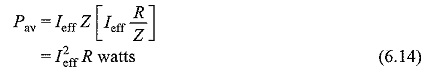This gives the average power dissipated in a resistive circuit.

If we consider a circuit consisting of a pure inductor, the power in the inductor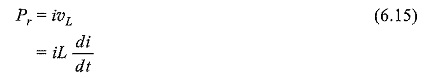Consider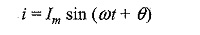Then

From the above equation, we can say that the average power delivered to the circuit is zero. This is called reactive power. It is expressed in volt-amperes reactive (VAR).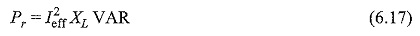From Fig. 6.4, we have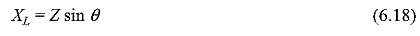Substituting Eq. 6.18 in Eq. 6.17, we ge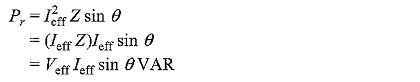### The Power Triangle:

A generalized impedance phase diagram is shown in Fig. 6.5. A phasor relation for power can also be represented by a similar diagram because of the fact that true power Pav and reactive power Pr differ from R and X by a factor I2eff, as shown in Fig. 6.5.The resultant power phasor I2eff Z, represents the apparent power Pa.

At any instant in time, Pa is the total power that appears to be transferred between the source and reactive circuit. Part of the apparent power is true power and part of it is reactive power.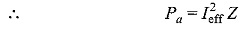The power triangle is shown in Fig. 6.6.

From Fig. 6.6, we can write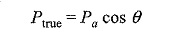or average power Pav = Pa cos θ
and reactive power Pr= Pa sin θ

Scroll to Top